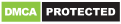# Electronics Toolkit

Genres
Version
-
Developer
-
Requires
-
Updated
-Electronics Toolkit is a helpful app with dozens of tools, calculators and references made for electronic engineers, students and hobbyists.

Calculators:
• Resistor color codes – calculate the resistance of resistors by selecting the colors of the bands

• SMD resistor codes – calculate the resistance of SMD resistors by entering the number

• LED resistor calculator – calculate the needed resistance to connect en LED to a power source

• Parallel resistors – calculate the resistance of resistors in parallel

• Voltage divider – calculate the output voltage of a voltage divider

• Series resistors – calculate the resistance of resistors in series

• Ohm’s law – calculate the voltage, current of resistance by entering the other two

• Capacitance calculator – calculate the capacitance, voltage or charge by entering the other two

• Battery discharge – calculate the time it takes to discharge a battery

• Inductor color codes – calculate the inductance of inductors by selecting the colors of the bands

• Parallel capacitors – calculate the capacitance of capacitors in parallel

• Series capacitors – calculate the capacitance of capacitors in series

• Unit converter – unit converter for length, temperature, area, volume, weight, time, angle, power and base

• Op-amp calculator – calculate the output voltage of non-inverting, inverting, summing and differential opamps

• Wheatstone bridge – calculate the resistance of one resistor in a balanced bridge or calculate the output voltage

• Inductor codes – calculate the inductance of inductors by entering the number

• Capacitor codes – calculate the capacitance of capacitors by entering the number

• DAC and ADC calculator – calculate the output of digital-analog and analog-digital converters

• Wavelength frequency calculator – calculate the frequency or wavelength of a wave

• SI prefixes – convert numbers with SI prefixes

• Capacitor energy – calculate the energy in an capacitor

• Slew rate calculator – calculate the slew rate

• Star delta transformation – calculate the resistors in a star delta transformation

• Zener calculator – calculate the resistance of the resistor and voltage of the zener

• Air core inductor calculator – calculate the inductance and wire length of an air core inductor

• 555 timer calculator – calculate the frequency, period, duty cycle, high time and low time of a popular 555 timer circuit

• Plate capacitor calculator – calculate the capacity of a plate capacitor

• Resistance to color code calculator – calculate the colors on the resistor by entering the resistance

• LM317 – calculate the output voltage of an LM317

• Low pass filters

• Wire resistance – calculate the resistance of electrical wire

• RMS voltage

• Decibel calculator

•Reactance

Tables:
• Logic gates – truth table of the 7 logic gates with interactive buttons

• 7-segment display – interactive display that you can change by clicking on one of the segments or by clicking on a button to show a hexadecimal character

• ASCII – decimal, hexadecimal, binary, octal and char ASCII table

• Resistivity – table with the resistivity of common metals at 293K

• Arduino pinout

• Pinout diagrams of 4000 and 7400 series ICs

Other:
• Bluetooth – connect to a bluetooth module like the HC-05 to talk with an arduino or other microcontroller with the terminal, button and slider modes

PERMISSIONS
• read the contents of your USB storage && modify or delete the contents of your USB storage – used to save images of IC pinout diagrams

• receive data from Internet && view network connections && full network access – used to load the IC data list and to collect statistics with Google Firebase

• pair with Bluetooth devices && access Bluetooth settings – used to connect with bluetooth devices

• prevent device from sleeping – prevents bluetooth devices from disconnecting
bug-fixes

Recommended for you

You may also like